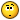Recommended Posts

Hello everybody,

before anything I'm going to introduce myself.

I am a Belgian Technical Artist working in the game/interactive software industry from some years now.

I was more a environment artist after my studies, but moved to development with Unity, Unreal and native C#/C++ software development.

I began to learn Houdini one year ago and having a developer background, it was more easy for me to understand how Vex is working.

I now try to use Houdini at work to improve our production pipeline with Unity and Unreal. Mostly using Vex. And it is a wonderful tool.

I am currently working on a river tool with heightfield and curves.

I first put the curve on top of the terrain then resample the curve and project each point on the terrain with the intersect function. (I also remove points that are outside the terrain)

`@P.y = getbbox_max(1).y + 100;`
```vector p, uvw;

int isIntersecting = intersect(1, @P, {0,-10000,0}, p, uvw);

if(isIntersecting == -1)
removepoint(0, @ptnum);
else
{
@N = @N;
@P.y = p.y;
}```

The problem is that a river always flow from top to bottom, but the projected curve on the terrain sometimes go up and down.

To fix that I loop through each points and check if the current point height (@P.y) is higher that the previous point height.

But it is not working as expected.

First try with Point Wrangle Run Over Points

```if(@ptnum > 0)
{
vector lastPointPosition = point(0,"P", @ptnum-1);

if(@P.y > lastPointPosition.y)
@P.y = lastPointPosition.y;
}```

Second try with Point Wrangle Run Over Detail(only once)

```int ptNumber = npoints(0);

for(int pt=1;pt<ptNumber;pt++)
{
vector currentPoint = point(0, "P", pt);
vector lastPoint = point(0, "P", pt-1);

float currentHeight = currentPoint.y;

//printf("%s %s\n", pt, pt-1);

if(currentHeight > lastPoint.y)
{
vector newPos = set(currentPoint.x, lastPoint.y, currentPoint.z);
setpointattrib(0, "P", pt, newPos, "set");
}
}```

What am I doing wrong ?Edited by PolygonExperiment

Share on other sites

Found a/the solution !

```int ptNumber = npoints(0);
vector currentPoint = {0,0,0};
vector lastPoint = point(0, "P", 0);

for(int pt=0;pt<ptNumber;pt++)
{
currentPoint = point(0, "P", pt);

if(currentPoint != 0 && lastPoint != 0 && currentPoint != lastPoint)
{
if(currentPoint.y > lastPoint.y)
{
//printf("%s\n", pt);
vector newPos = set(currentPoint.x, lastPoint.y, currentPoint.z);
setpointattrib(0, "P", pt, newPos, "set");
lastPoint = set(newPos);
}
else
{
lastPoint = currentPoint;
}
}
}```